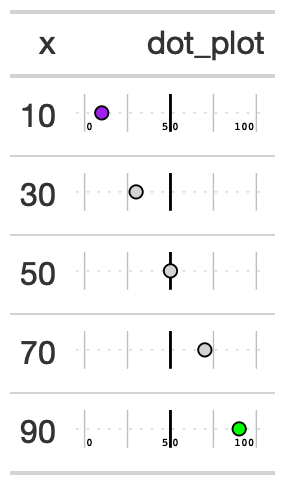Creates a percentile dot plot in each row. Can be used as an alternative for a 0 to 100% bar plot. Allows for scaling values as well and accepts a vector of colors for the range of values.

## Usage

gt_plt_percentile(
gt_object,
column,
width = 25,
scale = 1
)

## Arguments

gt_object

An existing gt table

column

The column to transform to the percentile dot plot. Accepts tidyeval. All values must be end up being between 0 and 100.

palette

A vector of strings of length 3. Defaults to c('blue', 'lightgrey', 'red') as hex so c("#007ad6", "#f0f0f0", "#f72e2e")

width

A numeric, indicating the width of the plot in mm, defaults to 25

scale

A number to multiply/scale the values in the column by. Defaults to 1, but can also be 100 if you have decimals.

a gt table

## Examples

library(gt)
dot_plt <- dplyr::tibble(x = c(seq(10, 90, length.out = 5))) %>%
gt() %>%
gt_duplicate_column(x,dupe_name = "dot_plot") %>%
gt_plt_percentile(dot_plot)

## Figures## Function ID

3-8

Other Plotting: gt_plt_bar_pct(), gt_plt_bar_stack(), gt_plt_bar(), gt_plt_dist(), gt_plt_point(), gt_plt_sparkline(), gt_plt_winloss()